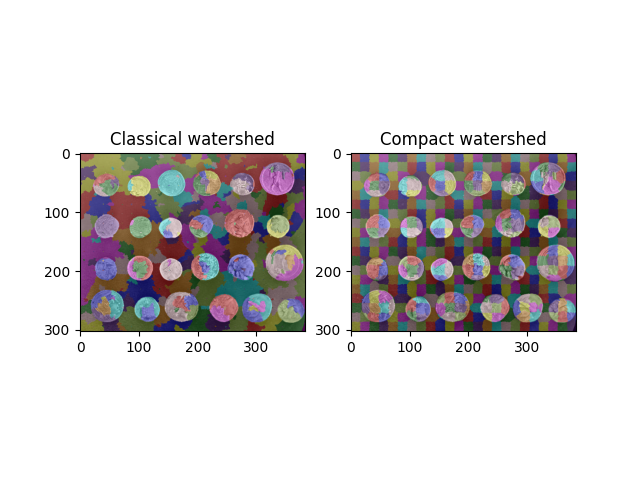# Find Regular Segments Using Compact Watershed¶

The watershed transform is commonly used as a starting point for many segmentation algorithms. However, without a judicious choice of seeds, it can produce very uneven fragment sizes, which can be difficult to deal with in downstream analyses.

The compact watershed transform remedies this by favoring seeds that are close to the pixel being considered.

Both algorithms are implemented in the `skimage.morphology.watershed()` function. To use the compact form, simply pass a `compactness` value greater than 0.```import numpy as np
from skimage import data, util, filters, color
from skimage.segmentation import watershed
import matplotlib.pyplot as plt

coins = data.coins()
edges = filters.sobel(coins)

grid = util.regular_grid(coins.shape, n_points=468)

seeds = np.zeros(coins.shape, dtype=int)
seeds[grid] = np.arange(seeds[grid].size).reshape(seeds[grid].shape) + 1

w0 = watershed(edges, seeds)
w1 = watershed(edges, seeds, compactness=0.01)

fig, (ax0, ax1) = plt.subplots(1, 2)

ax0.imshow(color.label2rgb(w0, coins, bg_label=-1))
ax0.set_title('Classical watershed')

ax1.imshow(color.label2rgb(w1, coins, bg_label=-1))
ax1.set_title('Compact watershed')

plt.show()
```

Total running time of the script: ( 0 minutes 0.167 seconds)

Gallery generated by Sphinx-Gallery